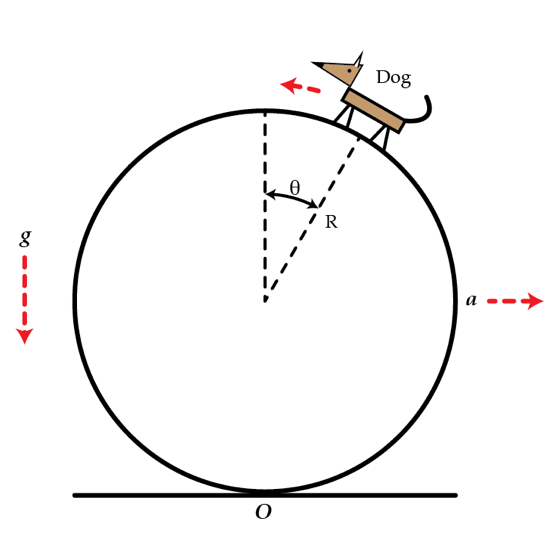# Circus dog

A small circus dog walks on a rolling solid cylinder. The dog remains at a fixed height above the ground corresponding to the angle $\theta= 30^{\circ}$. If the mass of the cylinder is 4 times larger than the mass of the dog, find the acceleration $a$ of the cylinder's center of mass in m/s$^2$. Assume that the cylinder rolls without slipping.Details and assumptions

• The acceleration of gravity is $-9.8~\mbox{m/s}^2$.
×

Problem Loading...

Note Loading...

Set Loading...